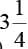# Olympiad Test: Simple Interest - 2

## 10 Questions MCQ Test Mathematics Olympiad Class 7 | Olympiad Test: Simple Interest - 2

Description
Attempt Olympiad Test: Simple Interest - 2 | 10 questions in 20 minutes | Mock test for Class 7 preparation | Free important questions MCQ to study Mathematics Olympiad Class 7 for Class 7 Exam | Download free PDF with solutions
QUESTION: 1

### What sum of the money will produce Rs 143 interest inyears at 2.5% simple interest?

Solution:

Let the required sum be Rs P.
Then, Rs P = (100 Rs 143)/ 3.25 × 2.5 = Rs 1760

QUESTION: 2

### A sum of money doubles itself in 10 years at simple interest. What is the rate of interest?

Solution:

Let the sum be Rs 100
After 10 years it becomes Rs 200
Therefore Interest = Rs 200 – Rs 100 = Rs 100
Then, Rate = (100 × I)/ (P × T)
= (100 × 100)/ (100 × 10)
= 10%

QUESTION: 3

### A sum of money trebles itself in 20 years at SI. Find the rate of interest.

Solution:

Rate = [100(3 – 1)]/20 = 10%

QUESTION: 4

In what time does a sum of money become four times at the simple interest rate of 5% per annum?

Solution:

Time
= [100(Multiple number of principal – 1)] / Rate
= [100(4 – 1)]/5 =60 years

QUESTION: 5

A sum was put at SI at a certain rate for 2 years. Had it been put at 3% higher rate, it would have fetched Rs 300 more. Find the sum.

Solution:

Sum
= (More Interest × 100)/ (Time × More Rate)
= (300 × 100)/ (2 × 3) = 5000

QUESTION: 6

A sum of money doubles itself in 7 years. In how many years will it become fourfold?

Solution:

Rate = [100(2 – 1)]/7 =100/7
Therefore Time = [100(4 – 1)]/ (100/7)
= 21 years

QUESTION: 7

The simple interest on Rs 1650 will be less than the interest on Rs 1800 at 4% simple interest by Rs 30. Find the time.

Solution:

We may consider that Rs (1800 – 1650) gives interest of Rs 30 at 4% per annum.
Therefore, Time = (30 × 100)/ (150 × 4) = 5 years

QUESTION: 8

Bobby invested a certain sum of money at 8% p.a. simple interest for ‘n’ years. At the end of ‘n’ years, Bobby got back 4 times his original investment. What is the value of n?

Solution:

Let us say Bobby invested Rs 100.
Then, at the end of ‘n’ years he would have got back Rs 400.
Therefore, the Simple Interest earned
= 400 – 100 = Rs 300.
Simple Interest = PRT/100
Substituting the values in the above equation of we get
300 = (100 × n × 8)/100
⇒ 8n = 300
⇒ n = 37.5 years.

QUESTION: 9

Anita invested a certain sum of money in a bank that paid simple interest. The amount grew to Rs 240 at the end of 2 years. She waited for another 3 years and got a final amount of Rs 300. What was the principal amount that she invested at the beginning?

Solution:

The sum grew to Rs 240 at the end of 2 years.
At the end of another 3 years, the sum grew to Rs 300
i.e. in 3 years, the sum grew by Rs 60
Therefore, each year, it grew by Rs 20
Sum at the end of 2 years = Rs 240
Sum grew by Rs 20 each year.
Hence, in the first 2 years, sum grew by 2 × 20 = Rs 40
Therefore, sum at the beginning of the period = Sum at the end of 2 years – Rs 40
= Rs 240 – Rs 40 = Rs 200

QUESTION: 10

Pratap invested a certain sum of money in a simple interest bond whose value grew to Rs 300 at the end of 3 years and to Rs 400 at the end of another 5 years. What was the rate of interest in which he invested his sum?

Solution:

Initial amount invested = Rs X
Therefore, the interest earned for the 5 years period between the 3rd year and 8th year = Rs 400 – Rs 300 = Rs 100
As the simple interest earned for a period of 5 years is Rs 100, interest earned per year = Rs 20
Therefore, interest earned for 3 years
= 3 × 20 = Rs 60
Hence, initial amount invested X
Amount after 3 years – interest for 3 years
= 300 – 60 = Rs 240
Rate of interest = (interest per year/ principal invested) × 100
= (20/240) × 100 = 8.33%Use Code STAYHOME200 and get INR 200 additional OFF Use Coupon Code